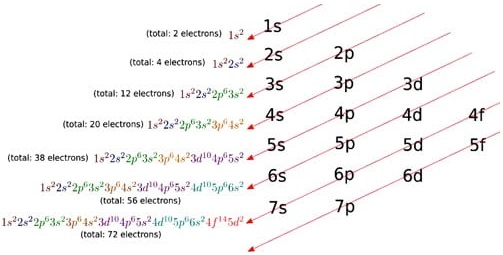Find free online Chemistry Topics covering a broad range of concepts from research institutes around the world.

## Electronic Configuration – Detailed Explanation with Examples

We have already learnt in XI STD to write the electronic configuration of the elements using Aufbu principle, Hund’s rule etc. According to Aufbau principle, the electron first fills the 4s orbital before 3d orbital. Therefore filling of 3d orbital starts from Sc, its electronic configuration is [Ar]3d14s2 and the electrons of successive elements are progressively filled in 3d orbital and the filling of 3d orbital is complete in Zinc, whose electronic configuration is [Ar]3d104s2.

However, there are two exceptions in the above mentioned progressive filling of 3d orbitals; if there is a chance of acquiring half filled or fully filled 3d sub shell, it is given priority as they are the stable configuration, for example Cr and Cu. The electronic configurations of Cr and Cu are [Ar] 3d54s1 respectively.

The extra stability of half filled and fully filled d orbitals, as already explained in XI STD, is due to symmetrical distribution of electrons and exchange energy. Note: The extra stability due to symmetrical distribution can also be visualized as follows. When the d orbitals are considered together, they will constitute a sphere.

So the half filled and fully filled configuration leads to complete symmetrical distribution of electron density. On the other hand, an unsymmetrical distribution of electron density as in the case of partially filled configuration will result in building up of a potential difference.To decrease this and to achieve a tension free state with lower energy, a symmetrical distribution is preferred. With these two exceptions and minor variation in certain individual cases, the general electronic configuration of d – block elements can be written as [Noble gas] (n – 1)d1-10 ns1-2

To calculate an electron configuration, divide the periodic table into sections to represent the atomic orbitals, the regions where electrons are contained. Groups one and two are the s-block, three through 12 represent the d-block, 13 to 18 are the p-block and the two rows at the bottom are the f-block.

Electronic configuration, also called electronic structure, the arrangement of electrons in energy levels around an atomic nucleus. In terms of a more refined, quantum-mechanical model, the K-Q shells are subdivided into a set of orbitals (see orbital), each of which can be occupied by no more than a pair of electrons.

There are different orbital shapes (s, p, d, f) Each orbital can only hold 2 electrons max. There is a hierarchy, i.e. s orbitals will be filled before p orbitals which will be filled before d orbitals and so on. (s<p<d<f) (note, this is a general rule but there are exceptions).

The electron configuration is the standard notation used to describe the electronic structure of an atom. When assigning electrons to orbitals, we must follow a set of three rules: the Aufbau Principle, the Pauli Exclusion Principle, and Hund’s Rule.

If you are given with the atomic number of an element you can find it’s period number and group number. The period number is related to the number of electron occupied shells in the element and the period number is linked to its valence electrons.

There are two main exceptions to electron configuration: chromium and copper. In these cases, a completely full or half full d sub-level is more stable than a partially filled d sub-level, so an electron from the 4s orbital is excited and rises to a 3d orbital.